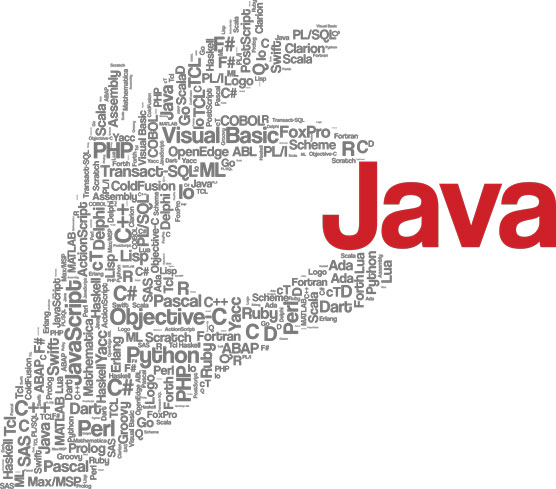##### Java All-in-One For Dummies, 6th EditionWriting Java statements (like `for `and `if`) and classes (like `Math` and `NumberFormat`) help you start and build strong programs. Variables hold different kinds of Java data types: numbers, characters, and true/false numbers. You designate Java operations that can be performed on operands, including arithmetic operators, relational operators (or binary) and logical operators (or Boolean).© DeymosHR/Shutterstock.com

## Common Java statements

Java statements build programs. Every Java class must have a body, which is made up of one or more statements. You can write different kinds of statements, including declaration and expression.

`break;`

### The continue statement

`continue;`

### The do statement

```do
{statements...}
while (expression);```

### The for statement

```for (init; test; count)
{statements...}```

### The enhanced for statement

```for (type variable : array-or-
collection)
{statements...}```

### The if statement

```if (expression)
{statements...}
else
{statements...}```

### The throw statement

`throw (exception)`

### The switch statement

```switch (expression)
{
case constant:
statements;
break;
default:
statements;
break;
}```

### The while statement

```while (expression)
{statements...}```

### The try statement

```try
{statements...}
catch (exception-class e)
{statements...}...
finally
{statements...}
try
{statements...}
finally
{statements...}```

## Primitive data types

Java data types are the kind of data you can store in a variable. Primitive data types are defined by the language itself. Java defines a total of eight primitive types. Of the eight primitive data types, six are for numbers, one is for characters, and one is for true/false values. Of the six number types, four are types of integers, and two are types of floating-point numbers.

Type Wrapper Class Parse Method of Wrapper Class
`int` `Integer` `int parseInt(String s)`
`short` `Short` `short parseShort(String s)`
`long` `Long<`/span> `long parseLong(String s)`
`byte` `Byte` `byte parseByte(String s)`
`float` `Float` `float parseFloat(String s)`
`double` `Double` `double parseDouble(String s)`
`char` `Character` (none)
`boolean` `Boolean` `boolean parseBoolean(String s)`

## Math and NumberFormat classes

Java classes lay the foundation for your programs. The Java `Math` and `NumberFormat` classes let you program number values, as well as format numbers and currencies.

The Math Class
Method Description
`num abs(num y);` Absolute value of y (num can be any numeric data type)
`num max(num y, num z);` Maximum of y and z
`num min(num y, num z);` Minimum of y and z
`double = Math. random();` Random number, such that 0.0 < x <= 1.0
The NumberFormat Class
Method Description
```NumberFormat getNumberInstance();``` Gets an instance that formats numbers.
`NumberFormat` Gets an instance that formats currency.
`String format(x);` Formats the specified number.

## Java operators

An operator designates a mathematical operation or some other type of operation that can be performed on operands. Java has arithmetic operators, relational operators (also known as binary operators) and logical operators (also known as Boolean operators).

Arithmetic
Operator Description
Subtraction
* Multiplication
/ Division
% Remainder
++ Increment
Decrement
-= Subtraction and assignment
*= Multiplication and assignment
/= Division and assignment
%= Remainder and assignment

Relational
Operator Description
== Equal
!= Not equal
< Less than
<= Less than or equal to
> Greater than
>= Greater than or equal to

Logical
Operator Description
! Not
& And
&& Conditional and
| Or
|| Conditional or
^ xor